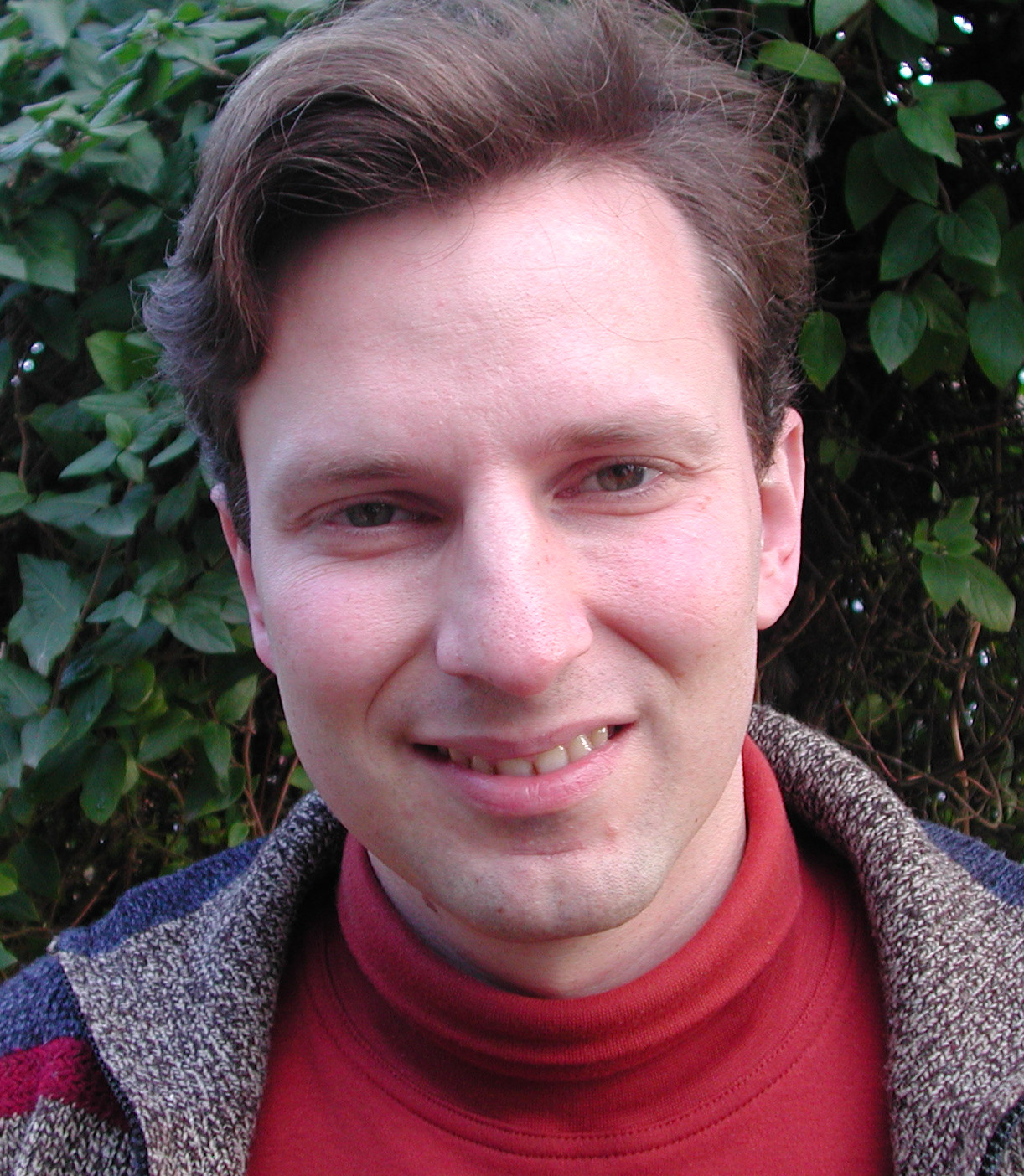# Invited Talks

## Titles and Abstracts

Ioannis Z. Emiris,
National Kapodistrian University of Athens and Athena Research Innovation Center### Compact formulae in sparse elimination

Abstract:

It has by now become a classic approach to use the theory of sparse (or toric) elimination, based on the Newton polytope of a polynomial, in order to reveal and exploit the structure of algebraic systems. This talk examines the notion of compact formula in view of standard and recent methods in sparse elimination.

We start with mixed volume, which bounds the number of toric roots of an algebraic system. We juxtapose closed-form expressions to generating functions in the case of semi-mixed multihomogeneous systems, and compare how far the two approaches can be extended. The most general systems admitting a closed formula have variables in a unique block structure and the Newton polytope per block is fixed but scaled independently per equation. Generating functions are available for sparse multihomogeneous systems arising in the computation of Nash equilibria.

For the sparse resultant, a bevy of results have established determinantal formulae for a large class of systems, starting with Macaulay. The discriminant is closely related to the resultant but admits no compact formula except for very simple cases. We offer a determinantal formula for the discriminant of the Nash equilibrium system, and illustrate the difficulty of the general question. We introduce an alternative notion of compact formula, namely the Newton polytope of the unknown polynomial. It is possible to compute it efficiently for sparse resultants, discriminants, as well as the implicit equation of a parameterized variety. Beyond hypersurfaces, the method extends to parameterized varieties of higher codimension, by means of the Chow form.

J. Ian Munro,
University of Waterloo### Succinct Data Structures ... Potential for Symbolic Computation?

Abstract: Succinct data structures are data representations that use the (nearly) the information theoretic minimum space for the combinatorial object they represent, while performing the necessary query operations in constant (or nearly constant) time. So, for example, we can represent a binary tree on n nodes in 2n+o(n) bits, rather than the “obvious” 5n or so words, i.e. 5n lg n bits. Such a difference in memory requirements can easily translate to major differences in runtime as a consequence of the level of memory in which most of the data resides. The field developed to a large extent because of applications in text indexing, so there has been a major emphasis on trees and a secondary emphasis on graphs in general; but in this talk we will draw attention to a much broader collection of combinatorial structures for which succinct structures have been developed, perhaps with potential for use in symbolic computation systems. These will include sets, permutations, functions, partial orders and groups, and yes, a bit on graphs.

Carsten Schneider,
RISC, Johannes Kepler University### Symbolic summation in difference rings and applications

Abstract:

Symbolic summation started with Abramov (1971) for rational sequences and has been pushed forward by Gosper (1978), Zeilberger (1991) and Paule (1995) to tackle indefinite and definite sums for hypergeometric expressions. In the last decade the class of input sums has been extended significantly and covers, for instance, hypergeometric multi-sums, holonomic sequences, unspecified sequences, radical expressions, Stirling numbers, etc.

In this talk we will focus on a new difference ring approach. The foundation was led by Karr's summation algorithm (1981) which enables one to rephrase indefinite nested sums and products in the setting of difference fields. Many new ideas have been incorporated into a strong summation theory which led to new algorithms for the summation paradigms of telescoping, creative telescoping and recurrence solving. However, this elegant difference field approach has one central drawback. Alternating signs cannot be represented in such a field: zero-divisors are introduced which can be formulated only within a ring. We will present a class of difference rings in which one can represent algorithmically indefinite nested sums and products together with the alternating sign, and more generally products over primitive roots of unity. In this setting we can represent all indefinite nested sums defined over hypergeometric expressions. In particular, this construction produces expressions in terms of sums that are all algebraically independent over each other. As a consequence, the derived output of a nested product-sum expression solves the zero-recognition problem: the computed expression evaluates to the zero-sequence if and only if the expression has been simplified to zero.

In combination with improved parameterized telescoping algorithms and recurrence solvers within such difference rings we obtain an efficient summation machinery that has been built into the summation package Sigma. We will illustrate the different summation techniques by large scale problems coming from the field of particle physics.